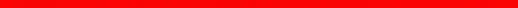Physics Department | Astronomy at UM |  Search

Review Checklist - Sample Questions

Main Properties of Stars

Distances

• What is parallax?
• For which stars can we find the distance using parallax?
• Out to what distance is parallax useful for stars?
• How is a parsec defined?
• How big is a parsec approximately?
• Which is the nearest star to us (other than the Sun)?
• How far is Proxima Centauri from us?
• Can we find the distance to Alpha Centauri using parallax?

Motion

• How do we measure radial motion of stars?
• What is proper motion?
• How can we measure a star's proper motion?
• Can we find the speed of a star without knowing its distance?
• Which star has the greatest proper motion, as seen from Earth?

Brightness and Other Properties

• What is the luminosity of a star?
• What is the magnitude of a star?
• What is the difference between absolute and apparent magnitude?
• Which is the brightest star in the sky (other than the Sun)?
• How can we find out the temperature of a star?
• How can we find out the size of a star?
• What can we measure without knowing the distance to a star?page by luca bombelli <bombelli at olemiss.edu>, modified 29 sep 2012